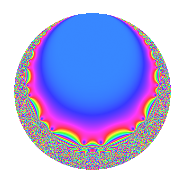# Properties

 Label 20.8.a.bLevel 20 Weight 8 Character orbit 20.a Self dual Yes Analytic conductor 6.248 Analytic rank 0 Dimension 2 CM No Inner twists 1

# Related objects

## Newspace parameters

 Level: $$N$$ = $$20 = 2^{2} \cdot 5$$ Weight: $$k$$ = $$8$$ Character orbit: $$[\chi]$$ = 20.a (trivial)

## Newform invariants

 Self dual: Yes Analytic conductor: $$6.24770050968$$ Analytic rank: $$0$$ Dimension: $$2$$ Coefficient field: $$\Q(\sqrt{1129})$$ Coefficient ring: $$\Z[a_1, a_2, a_3]$$ Coefficient ring index: $$2^{2}$$ Fricke sign: $$1$$ Sato-Tate group: $\mathrm{SU}(2)$

## $q$-expansion

Coefficients of the $$q$$-expansion are expressed in terms of $$\beta = 2\sqrt{1129}$$. We also show the integral $$q$$-expansion of the trace form.

 $$f(q)$$ $$=$$ $$q$$ $$+ ( -10 - \beta ) q^{3}$$ $$+ 125 q^{5}$$ $$+ ( 830 + 9 \beta ) q^{7}$$ $$+ ( 2429 + 20 \beta ) q^{9}$$ $$+O(q^{10})$$ $$q$$ $$+ ( -10 - \beta ) q^{3}$$ $$+ 125 q^{5}$$ $$+ ( 830 + 9 \beta ) q^{7}$$ $$+ ( 2429 + 20 \beta ) q^{9}$$ $$+ ( 1800 - 90 \beta ) q^{11}$$ $$+ ( 6590 + 36 \beta ) q^{13}$$ $$+ ( -1250 - 125 \beta ) q^{15}$$ $$+ ( 2730 + 468 \beta ) q^{17}$$ $$+ ( -20236 + 180 \beta ) q^{19}$$ $$+ ( -48944 - 920 \beta ) q^{21}$$ $$+ ( -20910 + 63 \beta ) q^{23}$$ $$+ 15625 q^{25}$$ $$+ ( -92740 - 442 \beta ) q^{27}$$ $$+ ( 59334 + 360 \beta ) q^{29}$$ $$+ ( -57964 + 3150 \beta ) q^{31}$$ $$+ ( 388440 - 900 \beta ) q^{33}$$ $$+ ( 103750 + 1125 \beta ) q^{35}$$ $$+ ( 153470 - 4536 \beta ) q^{37}$$ $$+ ( -228476 - 6950 \beta ) q^{39}$$ $$+ ( -176574 + 4860 \beta ) q^{41}$$ $$+ ( 607670 + 3195 \beta ) q^{43}$$ $$+ ( 303625 + 2500 \beta ) q^{45}$$ $$+ ( -1034250 + 153 \beta ) q^{47}$$ $$+ ( 231153 + 14940 \beta ) q^{49}$$ $$+ ( -2140788 - 7410 \beta ) q^{51}$$ $$+ ( 700230 - 13428 \beta ) q^{53}$$ $$+ ( 225000 - 11250 \beta ) q^{55}$$ $$+ ( -610520 + 18436 \beta ) q^{57}$$ $$+ ( -996252 - 10440 \beta ) q^{59}$$ $$+ ( -839338 - 36720 \beta ) q^{61}$$ $$+ ( 2828950 + 38461 \beta ) q^{63}$$ $$+ ( 823750 + 4500 \beta ) q^{65}$$ $$+ ( 1831970 + 25569 \beta ) q^{67}$$ $$+ ( -75408 + 20280 \beta ) q^{69}$$ $$+ ( 897468 + 18630 \beta ) q^{71}$$ $$+ ( 2531090 - 44604 \beta ) q^{73}$$ $$+ ( -156250 - 15625 \beta ) q^{75}$$ $$+ ( -2163960 - 58500 \beta ) q^{77}$$ $$+ ( 5089112 - 38340 \beta ) q^{79}$$ $$+ ( -2388751 + 53420 \beta ) q^{81}$$ $$+ ( -3607050 + 1143 \beta ) q^{83}$$ $$+ ( 341250 + 58500 \beta ) q^{85}$$ $$+ ( -2219100 - 62934 \beta ) q^{87}$$ $$+ ( -7665414 + 44280 \beta ) q^{89}$$ $$+ ( 6932884 + 89190 \beta ) q^{91}$$ $$+ ( -13645760 + 26464 \beta ) q^{93}$$ $$+ ( -2529500 + 22500 \beta ) q^{95}$$ $$+ ( 7012010 + 2124 \beta ) q^{97}$$ $$+ ( -3756600 - 182610 \beta ) q^{99}$$ $$+O(q^{100})$$ $$\operatorname{Tr}(f)(q)$$ $$=$$ $$2q$$ $$\mathstrut -\mathstrut 20q^{3}$$ $$\mathstrut +\mathstrut 250q^{5}$$ $$\mathstrut +\mathstrut 1660q^{7}$$ $$\mathstrut +\mathstrut 4858q^{9}$$ $$\mathstrut +\mathstrut O(q^{10})$$ $$2q$$ $$\mathstrut -\mathstrut 20q^{3}$$ $$\mathstrut +\mathstrut 250q^{5}$$ $$\mathstrut +\mathstrut 1660q^{7}$$ $$\mathstrut +\mathstrut 4858q^{9}$$ $$\mathstrut +\mathstrut 3600q^{11}$$ $$\mathstrut +\mathstrut 13180q^{13}$$ $$\mathstrut -\mathstrut 2500q^{15}$$ $$\mathstrut +\mathstrut 5460q^{17}$$ $$\mathstrut -\mathstrut 40472q^{19}$$ $$\mathstrut -\mathstrut 97888q^{21}$$ $$\mathstrut -\mathstrut 41820q^{23}$$ $$\mathstrut +\mathstrut 31250q^{25}$$ $$\mathstrut -\mathstrut 185480q^{27}$$ $$\mathstrut +\mathstrut 118668q^{29}$$ $$\mathstrut -\mathstrut 115928q^{31}$$ $$\mathstrut +\mathstrut 776880q^{33}$$ $$\mathstrut +\mathstrut 207500q^{35}$$ $$\mathstrut +\mathstrut 306940q^{37}$$ $$\mathstrut -\mathstrut 456952q^{39}$$ $$\mathstrut -\mathstrut 353148q^{41}$$ $$\mathstrut +\mathstrut 1215340q^{43}$$ $$\mathstrut +\mathstrut 607250q^{45}$$ $$\mathstrut -\mathstrut 2068500q^{47}$$ $$\mathstrut +\mathstrut 462306q^{49}$$ $$\mathstrut -\mathstrut 4281576q^{51}$$ $$\mathstrut +\mathstrut 1400460q^{53}$$ $$\mathstrut +\mathstrut 450000q^{55}$$ $$\mathstrut -\mathstrut 1221040q^{57}$$ $$\mathstrut -\mathstrut 1992504q^{59}$$ $$\mathstrut -\mathstrut 1678676q^{61}$$ $$\mathstrut +\mathstrut 5657900q^{63}$$ $$\mathstrut +\mathstrut 1647500q^{65}$$ $$\mathstrut +\mathstrut 3663940q^{67}$$ $$\mathstrut -\mathstrut 150816q^{69}$$ $$\mathstrut +\mathstrut 1794936q^{71}$$ $$\mathstrut +\mathstrut 5062180q^{73}$$ $$\mathstrut -\mathstrut 312500q^{75}$$ $$\mathstrut -\mathstrut 4327920q^{77}$$ $$\mathstrut +\mathstrut 10178224q^{79}$$ $$\mathstrut -\mathstrut 4777502q^{81}$$ $$\mathstrut -\mathstrut 7214100q^{83}$$ $$\mathstrut +\mathstrut 682500q^{85}$$ $$\mathstrut -\mathstrut 4438200q^{87}$$ $$\mathstrut -\mathstrut 15330828q^{89}$$ $$\mathstrut +\mathstrut 13865768q^{91}$$ $$\mathstrut -\mathstrut 27291520q^{93}$$ $$\mathstrut -\mathstrut 5059000q^{95}$$ $$\mathstrut +\mathstrut 14024020q^{97}$$ $$\mathstrut -\mathstrut 7513200q^{99}$$ $$\mathstrut +\mathstrut O(q^{100})$$

## Embeddings

For each embedding $$\iota_m$$ of the coefficient field, the values $$\iota_m(a_n)$$ are shown below.

For more information on an embedded modular form you can click on its label.

Label $$\iota_m(\nu)$$ $$a_{2}$$ $$a_{3}$$ $$a_{4}$$ $$a_{5}$$ $$a_{6}$$ $$a_{7}$$ $$a_{8}$$ $$a_{9}$$ $$a_{10}$$
1.1
 17.3003 −16.3003
0 −77.2012 0 125.000 0 1434.81 0 3773.02 0
1.2 0 57.2012 0 125.000 0 225.189 0 1084.98 0
 $$n$$: e.g. 2-40 or 990-1000 Significant digits: Format: Complex embeddings Normalized embeddings Satake parameters Satake angles

## Inner twists

This newform does not admit any (nontrivial) inner twists.

## Atkin-Lehner signs

$$p$$ Sign
$$2$$ $$-1$$
$$5$$ $$-1$$

## Hecke kernels

This newform can be constructed as the kernel of the linear operator $$T_{3}^{2}$$ $$\mathstrut +\mathstrut 20 T_{3}$$ $$\mathstrut -\mathstrut 4416$$ acting on $$S_{8}^{\mathrm{new}}(\Gamma_0(20))$$.